### Home > CCA > Chapter 9 > Lesson 9.3.1 > Problem9-74

9-74.
1. Thui made the following hypotheses: 2n − 1 < 5 and n + 1 ≤ 2n. Which of the following conclusions can she make? Homework Help ✎

1. n ≥ 1 and n ≤ 3

2. n ≥ 1 and n < 3

3. n > 1 and n ≤ 3

4. n > 1 and n < 3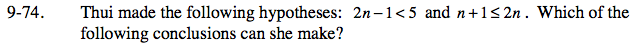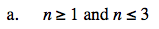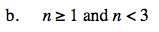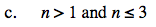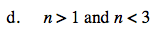Find the boundary points of the two equations.

2n − 1 = 5 n + 1 = 2n
2n = 6 1 = n
n = 3

Boundary points = 3 and 1

Test numbers on all sides of the boundary points.

Test n = 0
2(0) −1 < 5 0 +1 ≤ 2(0)
−1 < 5 1 ≤ 0
True False

Test n = 2
2(2) −1 < 5 2 +1 ≤ 2(2)
3 < 5 3 ≤ 4
True True

Test n = 4
2(4) +1 < 5 4 + 1 ≤ 2(4)
9 < 5 5 ≤ 8
False True

Because n = 2 is the only test that makes both equations true, (b), or 1 ≤ n < 3 is the correct answer.